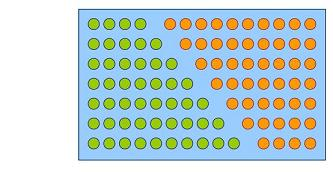#### You may also like### Diophantine N-tuples

Can you explain why a sequence of operations always gives you perfect squares?### DOTS Division

Take any pair of two digit numbers x=ab and y=cd where, without loss of generality, ab > cd . Form two 4 digit numbers r=abcd and s=cdab and calculate: {r^2 - s^2} /{x^2 - y^2}.### Sixational

The nth term of a sequence is given by the formula n^3 + 11n . Find the first four terms of the sequence given by this formula and the first term of the sequence which is bigger than one million. Prove that all terms of the sequence are divisible by 6.

# Reasonable Algebra

##### Age 14 to 16 Challenge Level:

Have you noticed that in the picture for 4+5+6+7+8+9+10 the rectangle has an even length and an odd heightHow about a diagram representing 8+9+10+11+12+13+14+15+16+17?
What can you say about the length and height of the rectangle in this case?

Is there a general rule?
Always?